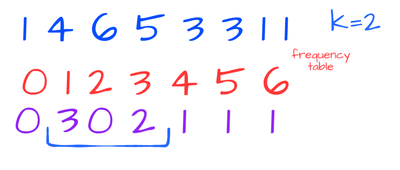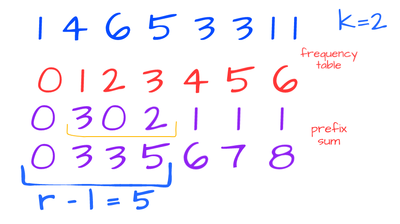# Picking Numbers

See the original problem on HackerRank.

## Solutions

### Sorting

A C++ solution based on sorting and a sliding window made of two increasing pointers:

  1 2 3 4 5 6 7 8 9 10 11 12 13 14 15 16 17 18 19 20 21 22 23 24 25 26 27 28 29 30 31 32 33 34 35 36  #include #include #include #include #include #include using namespace std; int main() { int n; cin >> n; vector v(n); copy_n(istream_iterator(cin), n, begin(v)); sort(begin(v), end(v)); auto selected = 0; auto tail = begin(v); auto head = next(tail); while (head != end(v)) { if (*head - *tail <= 1) { head++; } else { selected = max(selected, (int)distance(tail, head)); tail++; } } selected = max(selected, (int)distance(tail, head)); cout << selected; } 

Basically, we stretch out the windows as far as the difference between the endpoints is less than or equal to 1. When we find a greater difference, we calculate the distance of the pointers (the length of the window) so far and we increase the “tail” (the first endpoint). And so on. We just need to take extra care of the possible case where all the elements are equal. That’s handled on the line:

 1  selected = max(selected, (int)distance(tail, head)); 

### Frequency table

To avoid sorting and to maximize the performance when the size of the input grows a lot, an alternative solution takes advantage of the domain: indeed, adimissible numbers fall into the range $$[0-100]$$ and then we can do a statically sized array which stores all the occurrences. This is basically a way to “compress” the duplicates into a single element.

From the problem, we know that only adjacent elements in the occurences array (also known as frequency table) can be picked to maintain the constraint. So it’s just the matter of calculating the maximum among all the adjacent pairs:

  1 2 3 4 5 6 7 8 9 10 11 12 13 14 15 16 17 18 19 20 21 22  #include #include #include #include #include using namespace std; int main() { int n; cin >> n; array freq{}; while (cin >> n) freq[n]++; auto len = 0; for (auto i=1; i<100; ++i) { len = max(len, freq[i]+freq[i-1]); } cout << len; } 

A pattern brings from the solution: we applied the zip | map | reduce pipeline.

This pattern is easy to express in languages like Python:

  1 2 3 4 5 6 7 8 9 10 11 12 13  def pickingNumbers(l): freq=*100 for i in l: freq[i]+=1 zipped = zip(freq[1:], freq) mapped = map(lambda p: p+p, zipped) return reduce(max, mapped) if __name__ == "__main__": n = int(raw_input().strip()) a = map(int, raw_input().strip().split(' ')) result = pickingNumbers(a) print result 

Just for completeness, in C++ we can rewrite the solution in terms of** inner_product**:

  1 2 3 4 5 6 7 8 9 10 11 12 13 14 15 16 17 18 19 20 21 22  #include #include #include #include #include using namespace std; int main() { int n, i; cin >> n; array freq{}; while (cin >> i) { freq[i]++; } cout << inner_product(begin(freq), end(freq)-1, next(begin(freq)), 0, [](auto... args){ return max(args...); }, plus{}); } 

### Linq Solution by emanu_p81

  1 2 3 4 5 6 7 8 9 10 11  static int pickingNumbers(int[] a) { Dictionary frequencies = Enumerable.Range(0, a.Max() + 2) .ToDictionary(x => x, x => a.GroupBy(y => y) .Where(c => c.Key == x) .Select(f => f.Count()) .FirstOrDefault()); return frequencies .Zip(frequencies.Skip(1), (x, y) => (x.Value + y.Value)) .Max(); } 

## Another solution by alepez

  1 2 3 4 5 6 7 8 9 10 11 12 13 14 15 16 17 18 19 20 21 22 23  int pickingNumbers(vector a) { std::sort(begin(a), end(a)); auto l = begin(a); auto r = next(l); auto e = end(a); int currSize = 1; int maxSize = 1; while (r < e) { if (*r - *l <= 1) { ++currSize; } else { maxSize = max(currSize, maxSize); l = r; currSize = 1; } ++r; } return maxSize; } 

### C++ frequency table (alepez)

This is unreadable, but fits in 147 bytes of C++

 1 2 3  #include int main(){int f{};int n;while(std::cin>>n)++f[n];int m=0; for(int i=1;i!=100;++i)m=std::max(m,f[i-1]+f[i]);std::cout<

### ES6 frequency table + zip + reduce

 1 2 3 4 5 6 7 8 9  const zip = (arr, ...arrs) => arr.map((val, i) => arrs.reduce((a, arr) => [...a, arr[i]], [val])); const pickingNumbers = (a) => { let freq = Array(100).fill(0); a.forEach(n => ++freq[n]); return zip(freq, freq.slice(1)) .slice(0, -1) .reduce((max, p) => Math.max(max, p + p), 0); } 

### Generalization

It’s possible to generalize this problem to any absolute difference, not just 1. This change does not impact very much on the first solution because we just need to change the condition of the if:

 1  if (*head - *tail <= K) 

On the other hand, generalizing the second solution is much more interesting. When K=1 we have the original problem and we know that the solution is given by calculating the max among all the adjacent pairs aggregations. If K increases, then we still have to calculate the maximum of all the aggregations of adjacent windows of size K+1 (with K=1, windows are just pairs):We can do better. We can avoid aggregating numbers that we already aggregated in a previous step. One simple way to implement this is by maintaining an accumulator along the way and every time we move the window by one we subtract the element “leaving the window” and, at the same time, we add the element “entering the window”.

This is basically another pattern that we can implement in terms of the prefix sum: every element of the prefix sum in position $$i$$ is the sum of all the elements from the beginning to $$i$$. Then we can manage a window of size K+2 and subtracing the two endpoints along the way:The number of occurrences of the elements compressed in the frequency table will be just the difference between the two endpoints. So we can still apply the zip | map | reduce pattern just by changing the map:

  1 2 3 4 5 6 7 8 9 10 11 12 13 14 15 16 17 18 19 20 21 22 23  #include #include #include #include #include using namespace std; int main() { int n, i; cin >> n; array freq{}; while (cin >> i) { freq[i]++; } int k=1; partial_sum(begin(freq), end(freq), begin(freq)); cout << inner_product(next(begin(freq),k+1), end(freq), begin(freq), 0, [](auto...a){ return max(a...); }, minus<>{} ); } 
We've worked on this challenge in these gyms: modena  milan  padua  turin  bari